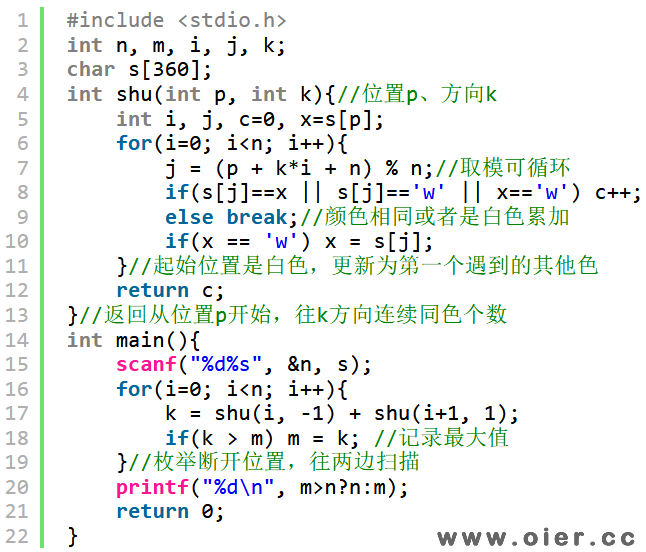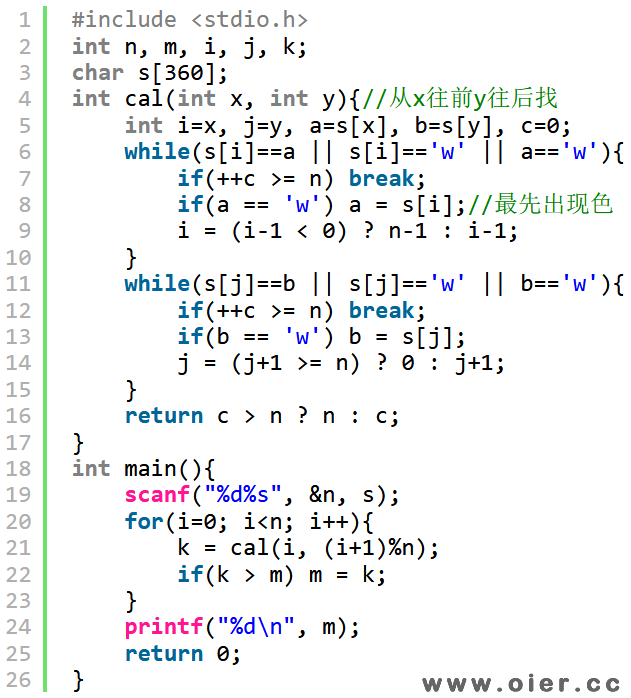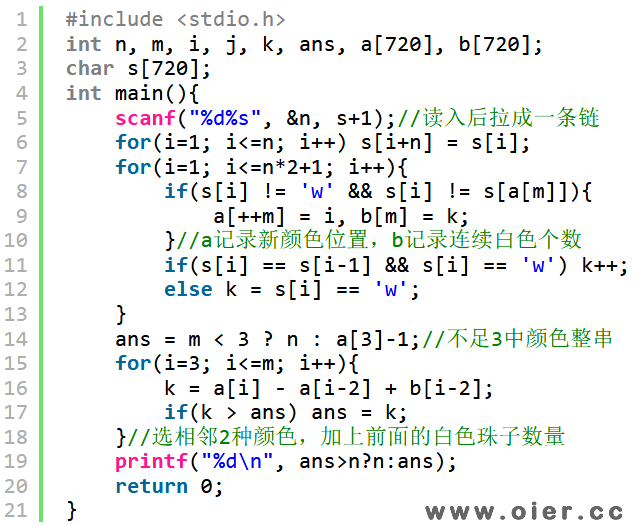SSOJ1069坏掉的项链[USACO]
1437+

题目描述

1 2                               1 2
r b b r                           b r r b
r         b                       b         b
r           r                     b           r
r             r                   w             r
b               r                 w               w
b                 b               r                 r
b                 b               b                 b
b                 b               r                 b
r               r                 b               r
b             r                   r             r
b           r                     r           r
r       r                         r       b
r b r                             r r w
图片 A                             图片  B

r 代表 红色的珠子
b 代表 蓝色的珠子
w 代表 白色的珠子

brbrrrbbbrrrrrbrrbbrbbbbrrrrb

输入

• 第 1 行: N, 珠子的数目
• 第 2 行: 一串长度为N的字符串, 每个字符是 r ， b 或 w。

样例输入

29
wwwbbrwrbrbrrbrbrwrwwrbwrwrrb

11

程序实现